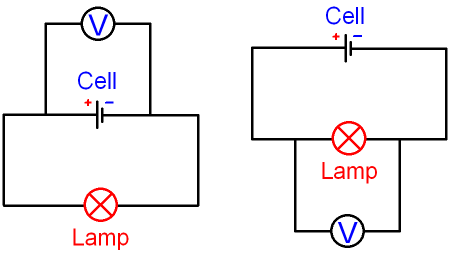gcsescience.com                                       5                                       gcsescience.com

Electricity

What is Potential Difference or Voltage?

Potential difference (or p.d.) is another word for voltage.
You can use either term to mean the same thing but most
exam questions use potential difference rather than voltage.

The potential difference (voltage) of a power supply is an
electrical pressure that causes a current to flow in a circuit.
Doubling the supply voltage will double the current.

What is a Volt?

The power supply (the cell or battery) gives an amount
of energy to each coulomb of charge in an electric circuit.

Energy is measured in joules.
A 6 volt cell gives 6 joules of energy to each coulomb.

1 volt = 1 joule per coulomb.

The word "per" means "divided by",
so  voltage = energy ÷ charge.

This equation can be rearranged to give
energy = voltage x charge.   E = V x Q.

Since Q = I x t, if we write I x t instead of Q
in the above equation we get E = V x I x t.
energy = voltage x current x time.

We can also use the word work
because work done = energy transferred

so you might see the equation
energy = voltage x charge    E = V x Q
written as
work = voltage x charge    W = V x Q

See all equations for electricity.

How is Potential Difference measured?

Potential difference is measured using a voltmeter.The circuit on the left shows the potential difference of the cell.
This is called the supply voltage. The circuit symbol
for a voltmeter is a circle with the letter V inside.
A voltmeter is always connected in parallel with a component.
The voltmeter is said to be connected across a component.
We use the word "across" to mean "in parallel with".

The circuit on the right has the voltmeter connected across
a lamp. This will tell you how many joules of energy are being
transferred from electrical energy into light energy (+ heat)
for each coulomb of charge that passes through the lamp.

A reading of 6 volts tells you that 6 joules of energy are
being transferred for each coulomb passing through the lamp.

A reading of 10 volts tells you that 10 joules of energy are
being transferred for each coulomb passing through the lamp.

gcsescience.com      Physics Quiz      Index      Electricity Quiz      gcsescience.com